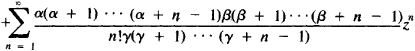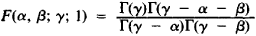# Hypergeometric Series

(redirected from Kummer series)

## hypergeometric series

[‚hī·pər‚jē·ə′me·trik ′sir·ēz]
(mathematics)
A particular infinite series which in certain cases is a solution to the hypergeometric equation, and having the form:
McGraw-Hill Dictionary of Scientific & Technical Terms, 6E, Copyright © 2003 by The McGraw-Hill Companies, Inc.
The following article is from The Great Soviet Encyclopedia (1979). It might be outdated or ideologically biased.

## Hypergeometric Series

a series of the form

F(α, β; γ; z) = 1The hypergeometric series was studied for the first time by L. Euler in 1778. Expansions of many functions into infinite series are special cases of hypergeometric series. For example,The hypergeometric series has meaning if γ is not equal to zero or a negative integer. It converges at |z| < 1. If, in addition, γ - α - β > 0, then the hypergeometric series also converges for z = 1. In this case, Gauss’ formula is valid:where Γ(z) is the gamma function. An analytic function, defined for |z| < 1 by a hypergeometric series is called a hypergeometric function and plays an important role in the theory of differential equations.